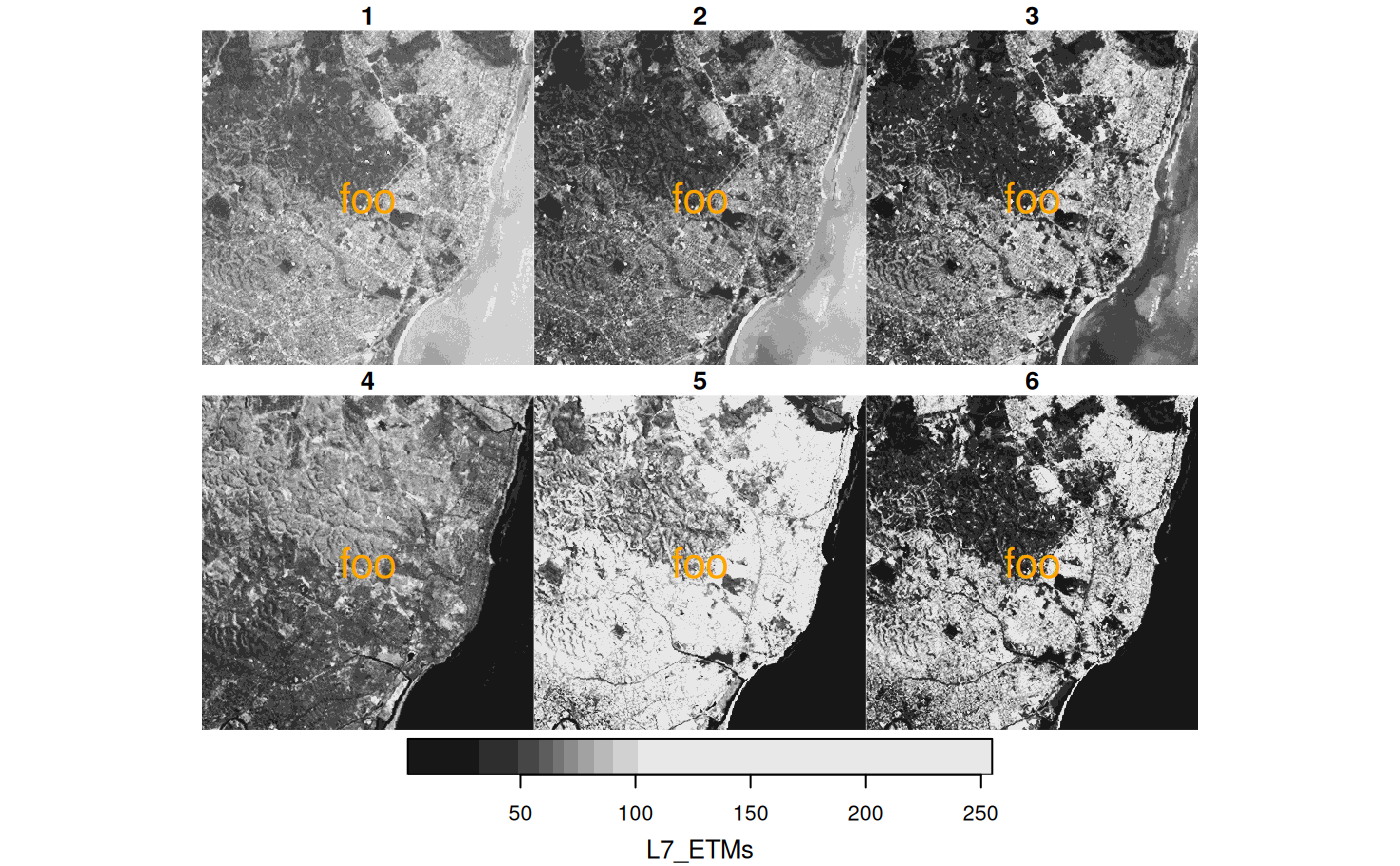plot stars object, with subplots for each level of first non-spatial dimension, and customization of legend key

# S3 method for stars
plot(
x,
y,
...,
join_zlim = TRUE,
main = make_label(x, 1),
axes = FALSE,
downsample = TRUE,
nbreaks = 11,
breaks = "quantile",
col = grey(1:(nbreaks - 1)/nbreaks),
key.pos = get_key_pos(x, ...),
key.width = lcm(1.8),
key.length = 0.618,
reset = TRUE,
box_col = grey(0.8),
center_time = FALSE,
hook = NULL,
mfrow = NULL
)

# S3 method for stars
image(
x,
...,
band = 1,
attr = 1,
asp = NULL,
rgb = NULL,
maxColorValue = ifelse(inherits(rgb, "data.frame"), 255, max(x[[attr]], na.rm =
TRUE)),
xlab = if (!axes) "" else names(d),
ylab = if (!axes) "" else names(d),
xlim = st_bbox(extent)$xlim, ylim = st_bbox(extent)$ylim,
text_values = FALSE,
text_color = "black",
axes = FALSE,
interpolate = FALSE,
as_points = FALSE,
key.pos = NULL,
logz = FALSE,
key.width = lcm(1.8),
key.length = 0.618,
border = NA,
useRaster = isTRUE(dev.capabilities()\$rasterImage == "yes"),
extent = x
)

# S3 method for nc_proxy
plot(x, y, ..., downsample = get_downsample(dim(x)), max_times = 16)

# S3 method for stars_proxy
plot(x, y, ..., downsample = get_downsample(dim(x)))

## Arguments

x

object of class stars

y

ignored

...

further arguments: for plot, passed on to image.stars; for image, passed on to image.default or rasterImage.

join_zlim

logical; if TRUE, compute a single, joint zlim (color scale) for all subplots from x

main

character; subplot title prefix; use "" to get only time, use NULL to suppress subplot titles

axes

logical; should axes and box be added to the plot?

downsample

logical or numeric; if TRUE will try to plot not many more pixels than actually are visible, if FALSE, no downsampling takes place, if numeric, the number of pixels/lines/bands etc that will be skipped; see Details.

nbreaks

number of color breaks; should be one more than number of colors. If missing and col is specified, it is derived from that.

breaks

actual color breaks, or a method name used for classIntervals.

col

colors to use for grid cells

key.pos

integer; side to plot a color key: 1 bottom, 2 left, 3 top, 4 right; set to NULL to omit key. Ignored if multiple columns are plotted in a single function call. Default depends on plot size, map aspect, and, if set, parameter asp.

key.width

amount of space reserved for width of the key (labels); relative or absolute (using lcm)

key.length

amount of space reserved for length of the key (labels); relative or absolute (using lcm)

reset

logical; if FALSE, keep the plot in a mode that allows adding further map elements; if TRUE restore original mode after plotting

box_col

color for box around sub-plots; use 0 to suppress plotting of boxes around sub-plots.

center_time

logical; if TRUE, sub-plot titles will show the center of time intervals, otherwise their start

hook

NULL or function; hook function that will be called on every sub-plot.

mfrow

length-2 integer vector with nrows, ncolumns of a composite plot, to override the default layout

band

integer; which band (dimension) to plot

attr

integer; which attribute to plot

asp

numeric; aspect ratio of image

rgb

integer; specify three bands to form an rgb composite. Experimental: rgb color table; see Details.

maxColorValue

numeric; passed on to rgb

xlab

character; x axis label

ylab

character; y axis label

xlim

x axis limits

ylim

y axis limits

text_values

logical; print values as text on image?

text_color

character; color for printed text values

interpolate

logical; when using rasterImage (rgb), should pixels be interpolated?

as_points

logical; for curvilinear or sheared grids: parameter passed on to st_as_sf, determining whether raster cells will be plotted as symbols (fast, approximate) or small polygons (slow, exact)

logz

logical; if TRUE, use log10-scale for the attribute variable. In that case, breaks and at need to be given as log10-values; see examples.

object of class sfc, or list with arguments to plot, that will be added to an image or sub-image

border

color used for cell borders (only in case x is a curvilinear or rotated/sheared grid)

useRaster

logical; use the rasterImage capabilities of the graphics device?

extent

object which has a st_bbox method; sets the plotting extent

max_times

integer; maximum number of time steps to attempt to plot.

## Details

Downsampling: a value for downsample of 0: no downsampling, 1: after every dimension value (pixel/line/band), one value is skipped (half of the original resolution), 2: after every dimension value, 2 values are skipped (one third of the original resolution), etc.

To remove unused classes in a categorical raster, use the droplevels function.

use of an rgb color table is experimental; see https://github.com/r-spatial/mapview/issues/208

when plotting a subsetted stars_proxy object, the default value for argument downsample will not be computed correctly, and has to be set manually.

when plotting a subsetted stars_proxy object, the default value for argument downsample will not be computed correctly, and has to be set manually.

## Examples

tif = system.file("tif/L7_ETMs.tif", package = "stars")